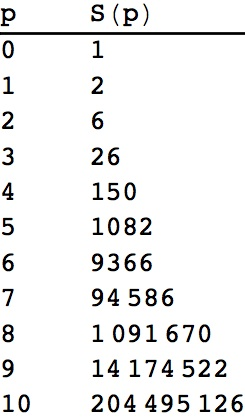## Questions about some infinite series and necklaces of partitions of labeled beads

We learn early in a study of infinite series that the geometric series$S=\sum_{k=1}^{\infty}1/2^k$ sums to 1.

Sometimes you will see this sort of reasoning:$S=1/2+1/4+1/8+ \ldots$ so$2S=1+(1+1/2+1/4+\ldots =1+S$ so$S=1$

which is somewhat suspect in light of Euler’s “argument”:$1+2+4+8+16+\ldots=1+2(1+2+4+8+\ldots)$ so$1+2+4+8+16+\ldots = -1$.

We need first to know that$S=\sum_{k=1}^{\infty}1/2^k$ converges absolutely, which we can do, for example by a use of the ratio test:

the$(k+1)^{\textrm{th}}$ term of$S=\sum_{k=1}^{\infty}1/2^k$ divided by the$k^{\textrm{th}}$ term is 1/2 <1.

Another application of the ratio test shows that the series$\sum_{k=1}^{\infty}k/2^k$ is absolutely convergent:

the$(k+1)^{\textrm{th}}$ of this series is$(k+1)/2^{k+1}$, the$k^{\textrm{th}}$ term is$k/2^k$ and their ratio is$\frac{(k+1)}{2^{k+1}}\frac{2^k}{k}=\frac{1}{2}(1+1/k)$ which approaches$1/2$ as$k \to \infty$.

If we denote$T=\sum_{k=1}^{\infty}k/2^k$ then a simple, and legitimate, calculation shows that$2T-T=1+1/2+1/4+1/8+\ldots$which we  know to be$1+1=2$.

In fact, for any natural number$p$ the ratio test shows that the series$S(p):=\sum_{k=1}^{\infty}k^p/2^k$ is absolutely convergent.

A Mathematica® calculation:

TableForm[Table[{p, Limit[Sum[k^p/2^k, {k, 1, n}], n -> Infinity]}, {p, 0, 10}], TableHeadings -> {None, {“p”, “S(p)”}}]

yields the following results for$S(p)$:The first curious thing is that the results are all whole numbers. Why is that?

The second curious thing is that if we enter this sequence of whole numbers into the The On-Line Encyclopedia of Integer Sequences we get a match:

these whole numbers match the number of necklaces of partitions of p+1 labeled beads. They also match the sequence of cumulants of the probability distribution of the number of tails before the first head in a sequence of fair coin tosses. Is that right? If so, why?$p=2$ is the first really interesting case.

A detailed account of these and similar series is dealt with by Mircea Cîrnu “Determinantal formulas for sum of generalized arithmetic-geometric series” Boletn de la Asociacion Matematica Venezolana, Vol. XVIII, No. 1 (2011), 13-25.

Enjoy! It’s connections like these that give mathematicians a buzz.